## 10 Questions MCQ Test Topicwise Question Bank for Electrical Engineering | Test: Sinusoidal Steady-State Analysis

Description
Attempt Test: Sinusoidal Steady-State Analysis | 10 questions in 30 minutes | Mock test for Electrical Engineering (EE) preparation | Free important questions MCQ to study Topicwise Question Bank for Electrical Engineering for Electrical Engineering (EE) Exam | Download free PDF with solutions
QUESTION: 1

### The phasor diagram shown in figure below is for a two-element series circuit having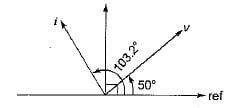Solution:

It is clear that i leads v by 53.2° (< 90°).
Hence the two series elements must be R and C.
∴ tan θ = tan 53.3°
tan 45° < tan 53.3° < tan 60° or,
1 < tan 53.3° <1.732
Hence, tan 53.3° = 1.3367

QUESTION: 2

### For the circuit shown below, the voltage across the 3 Ω resistor V3 is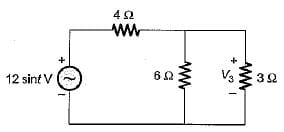Solution: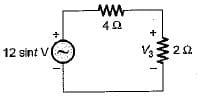Using voltage division rule, we have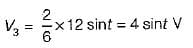QUESTION: 3

### A part of a circuit shown below consists of a resistor, a capacitor and an inductor. At steady state, iR(t) = 10 sin t and vL{t) = 5 cost. The rms value of the current through the capacitor is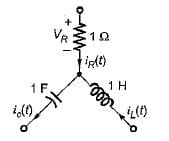Solution:

Using KCL at the given node,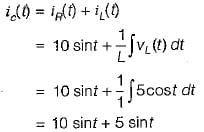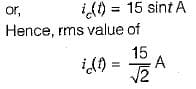QUESTION: 4

In the circuit shown below, currents I and I1 are respectively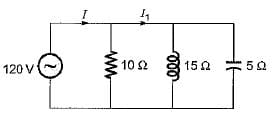Solution:

From given figure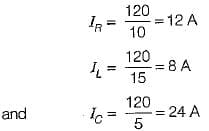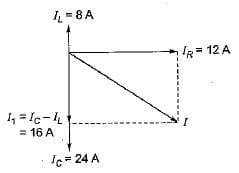Using phasor diagram shown below, the currents I and I1 are respectively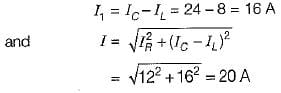QUESTION: 5

What should be the value of C for the circuit shown below such that the input power factor is unity for any frequency f of the source?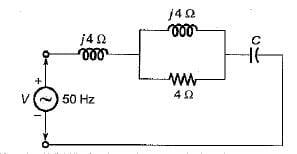Solution:

The input impedance Z of given circuit is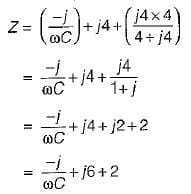For p.f. to be unity,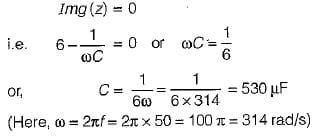QUESTION: 6

In a series R-L circuit, the current and voltages are given by
i = cos (314t-20°) , v = 10 cos ( 314 t + 10°). The values of R and L are respectively

Solution:

Here, i lags v by 30°

also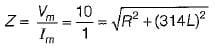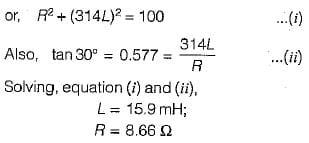QUESTION: 7

A series R-L circuit has resistance and reactance of 15 Ω and 10 Ω respectively. What should be the value of capacitor which when connected across the series combination in parallel, the system attains unity p.f.? (use f= 50 Hz)

Solution:

The situation is shown in figure below with it's phasor diagram.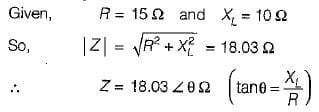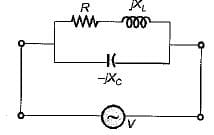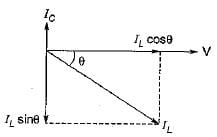For unity p.f. operation,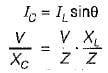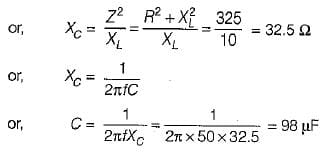QUESTION: 8

The input p.f. of the circuit shown below is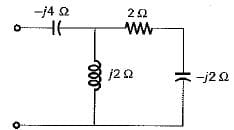Solution: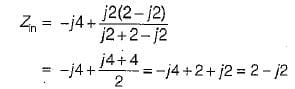or, Zin = 2.828∠-45°Ω
Hence, input p.f. = cos45° = 0.707 (lead)

QUESTION: 9

The input admittance of the circuit shown below is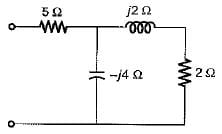Solution: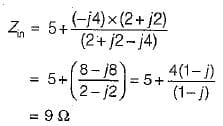Hence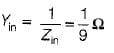QUESTION: 10

The driving point impedance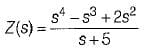does not represents a passive one port network because

Solution:Use Code STAYHOME200 and get INR 200 additional OFF Use Coupon Code